# Working with Dates in Flash

It's useful in Flash to be able to access date information to display the date, make a movie do specific things on certain dates, create countdown timers, or display the day of the week for a particular date in history, to name just a few examples.

To use dates in Flash, you create an instance of the Date class by using the following syntax:

` var myDate:Date = new Date(year, month, date); `

This syntax creates a new Date object named myDate. The parameters in parentheses associate the Date object with a specific date. For example:

` var myDate:Date = new Date(66, 6, 27); `

This example creates a Date object associated with July 27, 1966. The first parameter represents the year, the second represents the month, and the third represents the date. You may wonder why July, which we consider the seventh month in the year, is actually defined as the sixth month in the script above. In ActionScript, both months and days of the week are referenced by numbers, beginning with 0; therefore, January is the "zero" month, February is the first month, March is the second month, and so on up to December, which is the eleventh month. Likewise, days of the week begin with Sunday as the "zero" day, Monday the first day, and so on. The following exercise demonstrates the usefulness of this numbering system.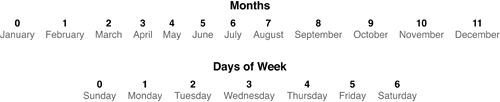NOTE

Dates and years are recognized by their true values; therefore, 66 refers to 1966, while 27 refers to the 27th day in the month.

When referencing years between 1900 and 1999, the year parameter needs only two digits the last two digits of the specified year (99 for 1999, 66 for 1966, and so on). Years outside these boundaries must be specified using all four digits (2007 for 2007, 2001 for 2001, and so on).

TIP

You can add parameters for the hour, minute, second, and millisecond if the application requires that precision.

To create a Date object that references the current point in time (as indicated by the user's system clock), you can leave the parameter settings blank:

` var myDate:Date = new Date(); `

After you've created the Date object, you can use Date class methods to retrieve all sorts of information about that date. For example, the preceding line of script would create a Date object that references today's date. To discover the current month, use the following syntax:

` var currentMonth:Number = myDate.getMonth(); `

If the current month is August, for example, currentMonth would have a value of 7 after executing.

To find out the current day of the week, use the following syntax:

` var currentDay:Number = myDate.getDay(); `

If the current day is Thursday (as set on the computer executing the script), currentDay would have a value of 4 after executing.

Other methods of the Date class allow you to retrieve information about the current hour, minute, and second, as defined by the user's computer clock. These methods include the following:

` myDate.getHours(); myDate.getMinutes(); myDate.getSeconds(); `

This functionality can be useful for displaying the current time in an application something we'll demonstrate later in this lesson.

TIP

Your project can contain multiple Date objects, each used for a different purpose.

#### Using the DateChooser Component

The DateChooser component provides an easily recognizable visual interface for displaying date-related information; in fact, it looks like a calendar. When placed in a project, the DateChooser component initially displays and highlights the current date according to the computer's system clock; but the DateChooser component does more than provide a pretty face for displaying the current date. It's also an interface for allowing the user to navigate dates. Dates can be selected by clicking them, and months can be navigated by clicking the left arrow icon (to move back one month) or the right arrow icon (to move forward one month).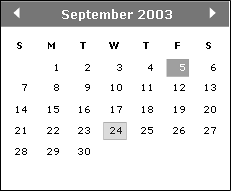With ActionScript, a DateChooser component instance can be moved instantly to a specific date, or information about a date that the user has manually highlighted can be retrieved and used in an application. Both of these tasks are made possible via the selectedDate property of DateChooser instances. This property's functionality relies on the use of Date objects, as discussed previously. Let's look at a couple of examples.

Suppose there's a DateChooser component instance named calendar_dc in your application. To tell this instance to move ahead to and display February 21, 2018, you use syntax like the following:

` var my25thAnniversary:Date = new Date(2018, 1, 21); calendar_dc.selectedDate = my25thAnniversary; `

The first line creates a Date object named my25thAnniversary, and the next line uses that Date object to set the value of the selectedDate property.

Here's a shorthand way of doing the same thing:

` calendar_dc.selectedDate = new Date(2018, 1, 21); `

When retrieving the current value of the selectedDate property, the component instance returns a Date object. The following example creates a Date object named displayedDate:

` var displayedDate:Date = calendar_dc.selectedDate; `

The date referenced by this object is the currently selected date in the calendar_dc component instance. Using methods of the Date class, you can retrieve information from this Date object (such as the selected day, month, and year), so that the application knows and can react to the date the user has selected.

In the following exercise, you'll create a highly interactive calendar application using Date objects, the setInterval() function, and an instance of the DateChooser component.

1. Open makeMyDay1.fla in the Lesson16/Assets folder.

This project contains three distinct sections: Calendar, Alarm, and Messages. In this exercise, we'll concentrate on making the Calendar section functional. The remaining sections are for later exercises.

The Calendar section consists of five text fields, a button, and a DateChooser component instance. The five text fields are shown in the following list (from top to bottom). These settings will be used to display various portions of the date dynamically:

• time_txt. Displays the current time in hours, minutes, and seconds. The result is updated for every second that time ticks away.

• calendarDay_txt. Displays the current day of the week (for example, Saturday).

• calendarDate_txt. Displays the current day of the month (for example, 27).

• calendarMonth_txt. Displays the current month (for example, April).

• calendarYear_txt. Displays the current year (for example, 2004).

The button is named showToday_btn and the DateChooser component instance is named calendar_dc.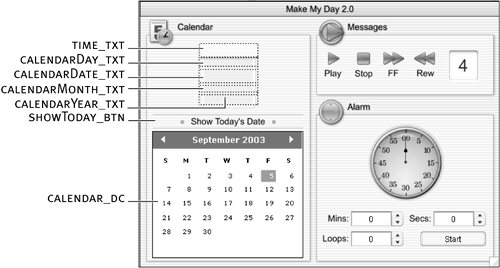When the application is opened and played initially, the text fields display the current date and time information. As the user interacts with the calendar_dc instance, the date information in the text fields is updated accordingly. Clicking the showToday_btn instance resets the calendar_dc instance and text field instances to display information related to today's date.

All the elements for this exercise reside on the Calendar Assets layer. Nearly all of the ActionScript for this project will be placed on Frame 1 of the Actions layer.

Our first task is to script the functionality for updating the time display, which shows the current hour, minute, and second as determined by the user's system clock. First we'll create a function that captures and displays the most up-to-date time information from the operating system; then we'll use the setInterval() action to call this function once every second.

2. With the Actions panel open, select Frame 1 on the Actions layer and add the following script:

` function updateTime(){ } `

In the next several steps, we'll add script to the updateTime() function that enables it to capture and display the current time.

3. Insert the following line of script within the updateTime() function:

` var currentTime = new Date(); `

This step creates a new Date object named currentTime. Because we haven't specified any date parameters within the parentheses, this Date object stores/references the current time on the user's computer that is, the exact time when the function is executed. The next several lines of script will extract the hour, minute, and second data within the object.

This Date object only needs to exist for the duration of this function's execution (as we'll demonstrate shortly); therefore, it has been declared as a local variable. Of course, if we needed it to exist beyond that point, we would have used strict typing syntax instead:

` var currentTime:Date = new Date(); `

4. Insert the following script after the script added in Step 3:

` var hour = currentTime.getHours(); var minute = currentTime.getMinutes(); var second = currentTime.getSeconds(); `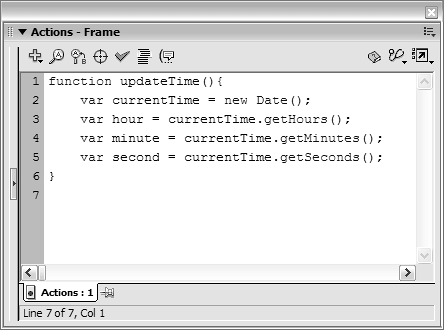These three lines of script create three variables named hour, minute, second. The values of these variables are determined by using various methods of the Date class:

• The getHours() method extracts the current hour from the currentTime Date object and assigns that value to the hour variable. The getHours() method always returns an hourly value based on what's commonly referred to as military time, where the 24 hours in a day are specified in a range from 0 to 23.

• The getMinutes() method extracts minute data from the currentTime Date object and assigns that value to the minute variable. The value returned by this method can be anything from 0 (indicating the top of the hour) to 59.

• The getSeconds() method is similar to the getMinutes() method. It returns a value between 0 and 59 (seconds); our script assigns that value to the second variable.

To help understand this functionality, let's look at an example. Suppose the current time on your computer is 4:04:07 p.m. If you execute the updateTime() function now, hour would be assigned a value of 16, minute a value of 4, and second a value of 7.

Although these values are accurate, there's an inherent formatting problem when displaying them in Flash: most clocks display time in the hour:minute:second format. If you plug in the current values of hour, minute, and second, the result is 16:4:7, which isn't easily understood to mean 4:04:07. The next task for our updateTime() function is to convert these raw time values into readable values that make sense to the general public. Hour values need to be converted to the 12-hour format; minute and second values need to add a beginning zero (0) if the value is less than 10.

5. Insert the following script after the script added in Step 4:

` var convertHour; if (hour >= 13){   convertHour = hour - 12; }else if(hour == 0){   convertHour = 12; }else{   convertHour = hour; } `

These eight lines of script convert the value of the hour variable from a military-time value into the more widely accepted 12-hour format. The converted value is stored in a variable named convertHour, which is created on the first line of the script. The conversion occurs as a result of the conditional statement. Here's the logic that went into putting it together.

As we mentioned earlier, the getHours() method always returns a value between 0 and 23, representing the hours between 12 a.m. and 11 p.m. 12 a.m. is represented by a value of 0 and 11 p.m. by a value of 23. Between 1 p.m. and 11 p.m., the hour variable would hold a value between 13 and 23. To reformat this value into the 12-hour format, we simply need to subtract 12 from it, which results in a new value between 1 and 11. Perfect! The first part of the conditional statement essentially says, "If hour has a value equal to or greater than 13, assign convertHour the result of subtracting 12 from hour." If hour has a value less than 13, this part of the statement is skipped and the next part evaluated.

The next part of the conditional statement handles what happens when hour has a value of 0 (representing 12 a.m.). In this case, convertHour is given a value of 12, which is an appropriate representation of 12 a.m. in the 12-hour format. If neither of the preceding conditions is true, the value of hour is already appropriate for the 12-hour format (3 a.m. is represented as 3, 10 a.m. as 10, and so on). In such a scenario, the value of hour is simply assigned to the value of convertHour. In the end, convertHour always has a value between 1 and 12.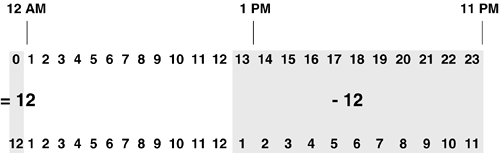6. Insert the following line after the conditional statement added in Step 5:

` var convertMinute = (minute < 10) ? "0" + minute : minute; `

This line of script adds "0" to minute values less than 10, so that numbers such as 2, 5, and 9 appear as 02, 05, and 09, respectively. Here we've used the ternary operator (?) to assign a converted minute value to the convertMinute variable. Because we haven't used the ternary operator that often, let's look at how it's used here.

The expression to the right of the equals sign could be read this way:

` (evaluate this expression) ? do this if true: do this if false; `

Our expression states: "If minute has a value less than 10, place a zero (0) in front of that value and assign the result to convertMinute. If the value of minute is greater than 10, assign the value of minute to convertMinute without doing anything." In the end, convertMinute has a value between 00 and 59.

7. Insert the following line after the script added in Step 6:

` var convertSecond = (second < 10) ? "0" + second : second; `

This line of script adds "0" to second values less than 10, in the same manner as the line of script discussed in Step 6.

Using our example of 4:04:07, after the conversion process convertHour has a value of 4, convertMinute a value of 04, and convertSecond a value of 07.

The last step we need to take is to string these values together, insert a colon (:) between them, and display the result in the time_txt text field.

8. Insert the following script after the script added in Step 7:

` var timeString = convertHour + ":" + convertMinute + ":" + convertSecond; time_txt.text = timeString; `

The first line of this script creates a variable named timeString that holds the concatenated values of convertHour, convertMinute, and convertSecond, separated by colons. The next line displays the resulting value of timeString in the time_txt text field.

This step completes the construction of the updateTime() function. The only thing left to do is get the application to call this function repeatedly, once every second.

9. Add the following line of script below the updateTime() function definition:

` setInterval(updateTime, 1000); `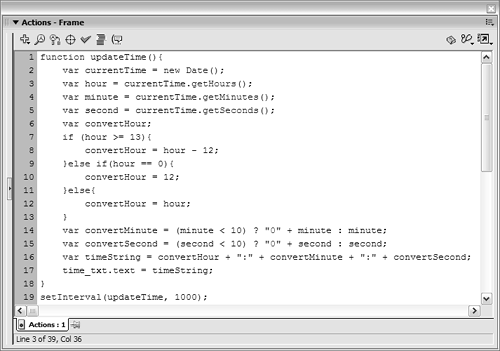This setInterval() action calls the updateTime() function once every second (1,000 milliseconds). Each time the updateTime() function is called, the current time data stored in the currentTime Date object is updated, extracted, converted, and displayed. The end result is an up-to-the-second time display. Let's check it out.

10. Choose Control > Test Movie.

As soon as the movie begins to play, the current time is displayed in the time_txt text field.

We still have a bit more work to do in this exercise.

11. Close the test movie to return to the authoring environment. With the Actions panel open and Frame 1 of the Actions layer selected, add the following script:

` function updateDate(eventObj:Object){ } calendar_dc.addEventListener("change", updateDate); `

The updateDate() function eventually will contain script telling the function how to display day, date, month, and year information in the text fields named calendarDay_txt, calendarMonth_txt, calendarDate_txt, and calendarYear_txt, respectively. The third line of the script above shows that we've set up the application to call the updateDate() function when the user selects a date (firing a change event) from the calendar_dc instance.

In addition to being called as a result of the change event, this function will be called under two other circumstances (which we have yet to script):

• When the application first opens

• When the showToday_btn instance is clicked

The function will display today's date information when the application is first opened and when the showToday_btn instance is pressed, but it will show the information for any date selected by the user when the user interacts with the calendar_dc instance. How will it know which of these actions to perform?

When called as a result of the user's interacting with the calendar_dc instance, the function is sent an Event object, as discussed in Lesson 10, "Scripting UI Components." Under the other two circumstances when the function is called, no Event is passed. The existence (or nonexistence) of this Event object is checked when the function is executed, allowing the function to react appropriately. This principle will become clearer in a moment.

12. Insert the following script within the updateDate() function:

` if(eventObj.target.selectedDate != undefined){   var calendarDate = calendar_dc.selectedDate; }else{   var calendarDate = new Date(); } `

This conditional statement helps the function determine what date information to display. The end result of this statement is a Date object named calendarDate that contains either today's date information or information related to the currently selected date in the calendar_dc instance. Let's look at how it works.

When the function is called as a result of the user's interacting with the calendar_dc instance, an Event object is sent to the function; the Event object contains a target property value of calendar_dc. The following expression:

` eventObj.target.selectedDate != undefined `

looks for the existence of a selectedDate property on eventObj.target, or the calendar_dc instance. When the function is called as a result of the user's interacting with the calendar_dc instance, this expression evaluates to true. When this function is called at any other time, no Event object is passed to the function, and the expression evaluates to false.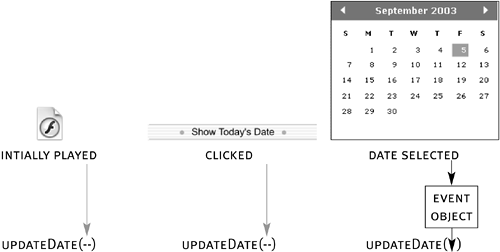When the expression evaluates to true, the value of calendar_dc.selectedDate is assigned to calendarDate, placing a Date object representing the currently selected date into calendarDate. If the currently selected date in the calendar_dc instance is July, 27, 2008, for example, the following line of script:

` var calendarDate = calendar_dc.selectedDate; `

is essentially the same as this one:

` var calendarDate = new Date(2008, 6, 27); `

The else part of the statement in Step 12 is executed when the function is called by any means other than the user's interacting with the calendar_dc instance. When this occurs, a Date object representative of today's date is placed into calendarDate.

The information contained in the calendarDate Date object plays a significant role in how the rest of the function works. The remainder of the function deals with extracting date information from the calendarDate Date object and displaying it in the appropriate text fields.

13. Insert the following lines of script after the conditional statement added in Step 12:

` var nameOfDays = ["Sunday", "Monday", "Tuesday", "Wednesday", "Thursday", "Friday",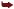"Saturday"]; var nameOfMonths = ["January", "February", "March", "April", "May", "June", "July","August", "September", "October", "November", "December"]; `

The script creates two arrays: nameOfDays and nameOfMonths. Each array contains several string values representing the names of days and months, respectively. These arrays are necessary because ActionScript doesn't assign names to months and days of the week. Instead, it references with a number the aspects of dates that we normally associate with a name. The trick to making this part of the project work is associating the proper name (based on its index value in the array) with the numbers ActionScript returns as the current day and month, respectively.

14. Insert the following line of script after the script added in Step 13:

` calendarDay_txt.text = nameOfDays[calendarDate.getDay()]; `

This action sets the information displayed in the calendarDay_txt text field. Here's how it works.

The getDay() method (inside the brackets) determines the day of the week for the calendarDate Date object. This method returns a value between 0 and 6, with 0 representing Sunday and 6 representing Saturday. If this method determines that the day of the week is 3, for example, that value is inserted into the brackets within the expression, making it look like this:

` calendarDay_txt.text = nameOfDays; `

At this point, calendarDay_txt displays the value residing at index position 3 of the nameOfDays array: the string value "Wednesday". Knowing that the getDay() method returns a value between 0 and 6, we created an array (in Step 13) with the names of the days at corresponding array index positions of 0 through 6. When the script is executed, the appropriate day name is used based on the numerical value returned by the getDay() method.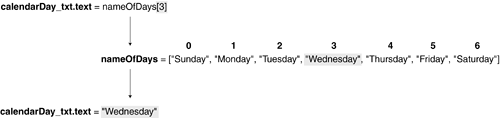15. Insert the following line of script after the line added in Step 14:

` calendarMonth_txt.text = nameOfMonths[calendarDate.getMonth()]; `

This action sets the information displayed in the calendarMonth_txt text field. It works like the action in Step 14, except that the getMonth() method returns a numerical value between 0 and 11 (for January through December). The nameOfMonths array from Step 13 has appropriate string values at corresponding index positions.

16. Insert the following line of script after the line added in Step 15:

` calendarDate_txt.text = calendarDate.getDate(); `

This action sets the information displayed in the calendarDate_txt text field. It uses the getDate() method, which returns a numerical value between 1 and 31, depending on the date associated with the Date object. Because we want to use the numerical value returned in this circumstance, we don't need to use an array to convert the information to a string value (as discussed in the previous two steps).

17. Insert the following line of script after the line added in Step 16:

` calendarYear_txt.text = calendarDate.getFullYear(); `

This action sets the information displayed in the calendarYear_txt text field. It uses the getFullYear() method, which returns a numerical value representing the full year of the date associated with the Date object (for example, 2003). Because we want to use the numerical value returned in this circumstance, we don't need to use an array to convert it to a string value.

This completes the function definition. As scripted in Step 11, this function is called when the user interacts with the calendar_dc instance (the change event). We still have to add several lines of script to call the function when the application is first opened or when the showToday_btn instance is clicked.

18. Add the following script after the line calendar_dc.addEventListener("change", updateDate);:

` updateDate(); showToday_btn.onRelease = function(){   calendar_dc.selectedDate = new Date();   updateDate(); } `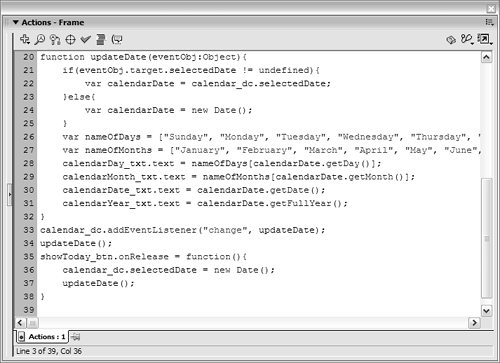The first line here calls the updateDate() function when the application is opened. The remaining lines assign an onRelease event handler to the showToday_btn instance. When this event is triggered, the script uses a new Date object representative of today to set the selectedDate property of the calendar_dc instance. This causes today's date to be displayed in the instance, no matter what date the user has navigated to. The second line within the event handler calls the updateDate() function.

Remember that neither of the two calls to the updateDate() function shown here sends an Event object to the function. As a result, the function is programmed to display today's date information automatically.

19. Choose Control > Test Movie to view the project to his point.

As soon as the movie appears, the text fields in the calendar section of the screen are populated with the appropriate data, displaying the full date. As you select dates from the calendar_dc instance, the date information is updated accordingly. Clicking the showToday_btn instance moves the calendar_dc instance to today's date and updates the displayed date information accordingly.

20. Close the test movie and save the project as makeMyDay2.fla.

We'll continue building on this file in the exercises that follow.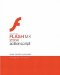Macromedia Flash MX 2004 ActionScript: Training from the Source
ISBN: 0321213432
EAN: 2147483647
Year: 2005
Pages: 182

Similar book on Amazon

flylib.com © 2008-2017.
If you may any questions please contact us: flylib@qtcs.net